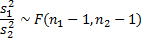# F Distribution

The F-distribution is primarily used to compare the variances of two populations, as described in Hypothesis Testing to Compare Variances. This is particularly relevant in the analysis of variance testing (ANOVA) and in regression analysis.

Definition 1: The The F-distribution with n1, n2degrees of freedom is defined byTheorem 1: If we draw two independent samples of size n1 and n2 respectively from two normal populations with the same variance thenProof: By Theorem 2 of Chi-square Distribution, If x is drawn from a normally distributed population N(μ ,σ) then for samples of size n:Thus if we draw two independent samples from two normal populations with the same variance σ, then by Definition 1,Property 1: A random variable t has distribution T(k) if and only if t2 has distribution F(1, k).

Excel Functions: The following Excel functions are defined for the  distribution:

FDIST(x, df1, df2) = the probability that the F-distribution with df1 and df2 degrees of freedom is ≥ x; i.e. 1 – F(x) where F is the cumulative F-distribution function.

FINV(α, df1, df2) = the value x such that FDIST(x, df1, df2) = 1 – α; i.e. the value x such that the right tail of the F-distribution with area α occurs at x. This means that F(x) = 1 – α, where F is the cumulative F-function.

With Excel 2010/2013/2016 there are a number of new functions (F.DIST, F.INV, F.DIST.RT and F.INV.RT) that provide equivalent functionality to FDIST and FINV, but whose syntax is more consistent with other distribution functions. These functions are described in Built-in Statistical Functions.

Observation: Excel only calculates the above functions for positive integer values of df1 and df2. Non-integer values are rounded down to the nearest integer. Thus, F.DIST(3,1.6,5,TRUE) = F.DIST(3,1,5,TRUE). In particular, all of the above Excel functions yield an error value when df1 < 1 or df2 < 1.

If you need a more accurate value of any of the F distribution functions when either or both of the degrees of freedom are not integers, and in particular when either of them is less than one, then you can use Real Statistics’ noncentral F distribution functions (with noncentrality value of zero), as described in Noncentral F Distribution. For example, the formula F.DIST(3,1,5,TRUE) = .8562, but F.DIST(3,0.99,5,TRUE) = #NUM!, whereas NF_DIST(3,0.99,5,0,TRUE) = .8606.

Alternatively, you can use the following Real Statistics functions.

Real Statistics Functions: The Real Statistics Resource Pack provides the following functions:

F_DIST(xdf1, df2, cum) = BETA.DIST(x * df1 / (x * df1 + df2), df1 / 2, df2 / 2, cum)

F_INV(pdf1, df2) = x * df2 / (df1 * (1 – x)) where x = BETA.INV(pdf1/2, df2/2)

Here F_DIST is a substitute for F.DIST and F_INV is as substitute for F.INV. Not only do these functions provide better estimates of the F distribution when the degrees of freedom are not integers, but F_DIST is also useful in providing an estimate of the pdf for versions of Excel prior to Excel 2010, where F.DIST(xdf1, df2, FALSE) is not available.

The Real Statistics Resource also provides the following functions:

F_DIST_RT(x, df1, df2) = 1 – F_DIST(x, df1, df2 TRUE)

F_INV_RT(p, df1, df2) = 1 – F_INV(p, df1, df2)

Statistics for Beginners – Discrete Probability Distributions

# Personal Career & Learning Guide for Data Analyst, Data Engineer and Data Scientist

## Applied Machine Learning & Data Science Projects and Coding Recipes for Beginners

A list of FREE programming examples together with eTutorials & eBooks @ SETScholars

# Projects and Coding Recipes, eTutorials and eBooks: The best All-in-One resources for Data Analyst, Data Scientist, Machine Learning Engineer and Software Developer

Topics included: Classification, Clustering, Regression, Forecasting, Algorithms, Data Structures, Data Analytics & Data Science, Deep Learning, Machine Learning, Programming Languages and Software Tools & Packages.
(Discount is valid for limited time only)`Disclaimer: The information and code presented within this recipe/tutorial is only for educational and coaching purposes for beginners and developers. Anyone can practice and apply the recipe/tutorial presented here, but the reader is taking full responsibility for his/her actions. The author (content curator) of this recipe (code / program) has made every effort to ensure the accuracy of the information was correct at time of publication. The author (content curator) does not assume and hereby disclaims any liability to any party for any loss, damage, or disruption caused by errors or omissions, whether such errors or omissions result from accident, negligence, or any other cause. The information presented here could also be found in public knowledge domains.`

# Learn by Coding: v-Tutorials on Applied Machine Learning and Data Science for Beginners

Please do not waste your valuable time by watching videos, rather use end-to-end (Python and R) recipes from Professional Data Scientists to practice coding, and land the most demandable jobs in the fields of Predictive analytics & AI (Machine Learning and Data Science).

The objective is to guide the developers & analysts to “Learn how to Code” for Applied AI using end-to-end coding solutions, and unlock the world of opportunities!